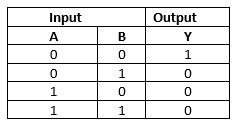# Logic Gates

## Logic Gates

• A logic gate is an digital circuit which follows logical relationship between input and output voltages
• It has one output and one or more inputs
• They are the basic building blocks from which most of the digital systems are build up
• They are called logic gates as they control the flow of information

• The five common logic gates used are NOT, AND, OR, NAND, NOR.
• Each logic gate is indicated by a symbol and its function is defined by a truth table that shows all the possible input logic level combinations with their respective output logic levels.
• Truth tables help understand the behaviour of logic gates.

## AND gate

• This gates has two or more input and one output. The output Y of AND gate is 1 only when both the input A and B are 1.
• Here is the logical symbol of AND gate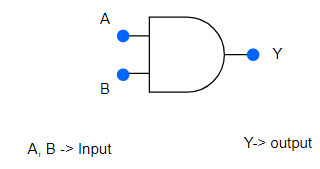• Truth Table is given below as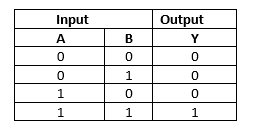## NOT gate

• This gates has one input and one output. This is most simplest gate. It produces a '1' output if the input is '0' and vice-versa.
• It is also called inverter
• Here is the logical symbol of NOT gate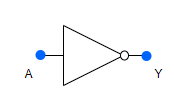• Truth Table is given below as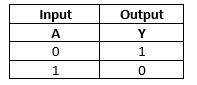## OR gate

• This gates has two or more input and one output. The output Y of OR gate is 1 when either input A or input B or both are 1s
• Here is the logical symbol of OR gate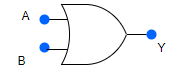• Truth Table is given below as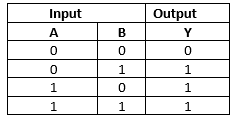## NAND gate

• This is AND gate followed by NOT gate. If inputs A and B are both '1', the output Y is not '1'.
• The gate gets its name from this NOT AND behaviour
• This is also called universal gate as all other gates can be created from it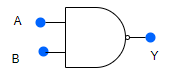• Truth Table is given below as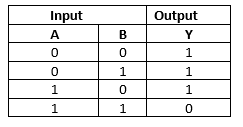## NOR gate

• This is OR gate followed by NOT gate. Its output Y is '1' only when both inputs A and B are '0'
• The gate gets its name from this NOT OR behaviour
• This is also called universal gate as all other gates can be created from it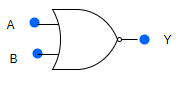• Truth Table is given below as